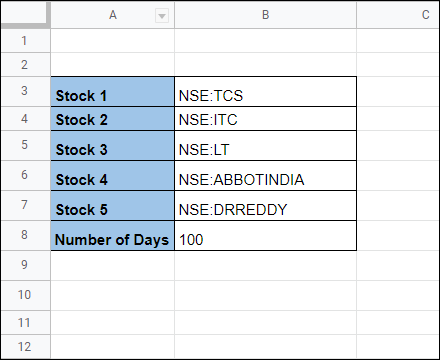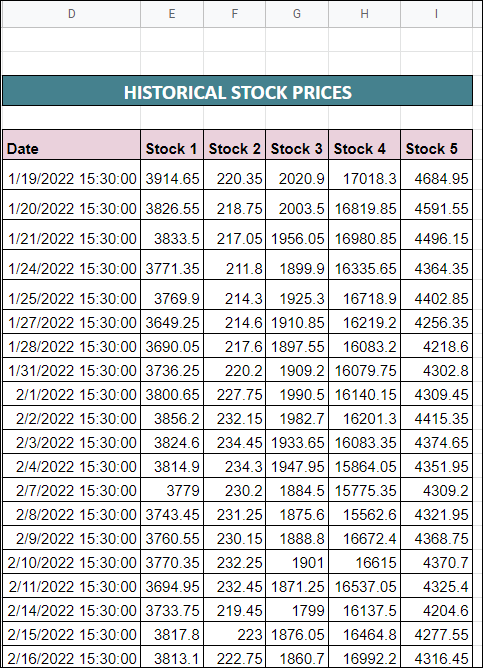# Correlation Matrix of Stocks in a Spreadsheet – Automatic Data Feed

Correlation Matrix is one of the great tools to balance and diversify your portfolio. It’s important that you don’t end up buying stocks that are highly correlated to each other, but to do that, you’ll need to know the exact correlation between stock prices. In this post, you’ll learn how to calculate the correlation matrix between different stocks in a simple spreadsheet.

But let’s first understand what is Correlation and Correlation matrix…

## What is a Correlation Matrix?

As the name suggests, correlation is a numeric figure (between -1 to 1) that tells you how strongly the two stocks are related to each other. Correlation can be applied to anything, but in this context, we are talking about stock prices.

• 1 indicates a strong positive relationship.
• -1 indicates a strong negative relationship.
• A value of zero indicates no relationship at all.

For example:

If Stock B moves by 10 points for every 10 point movement in Stock A, then the correlation between them is absolute 1.

If Stock B moves by 5 points for every 10 point movement in Stock A, then the correlation between them is 0.5.

If Stock B moves by -5 points for every 10 point movement in Stock A, then the correlation between them is -0.5.

When correlation is plotted in a row-column matrix like structure, then the resulting figure is termed a correlation matrix. It is an indispensable tool to summarize a large dataset and serves as a visual aid to identify similarities/dissimilarities in the data.

We’ll see an example of a correlation matrix later in this article.

Also Read: Sharpe Ratio Calculator Excel Sheet

## How is Correlation Calculated?

Correlation is calculated using the standard deviation and covariance.

It is the ratio of covariance between two variables and the product of the standard deviation of each one of them.

Cxy = Sxy / Sx*Sy

Sx and Sy are the sample standard deviations, and Sxy is the sample covariance.

I am sure this is something we all studied in our high school mathematics or statistics classes.

Any modern spreadsheet tools like MS Excel, Google Sheets, etc have a simple formula for creating correlation, and we are going to use the same for creating the stocks correlation matrix.

It’s a simple spreadsheet created using Google Sheets that fetches the historical prices of stocks using google finance and calculates correlations between them.

You can access the sheet from the below link:

To use this spreadsheet you’ll only need to enter the Stock symbols and number of historical days in Cells B3 to B8.Based on what you enter, the sheet will fetch the historical prices from Google finance in columns D to IAnd then based on these historical prices, the correlation matrix is calculated at columns K to PIf you click on any cell within the correlation matrix, you will find the below formula that is used to calculate the correlation coefficient

`=correl(\$E:\$E,F:F)`

The formula takes two inputs:

Input 1: Array of values for Stock 1

Input 2: Array of values for Stock 2

Depending on the correlation value the matrix is color-coded. The color ranges from Dark Red (for -1) to Dark Green (for +1)

Play around with the spreadsheet, try changing the stock symbols and historical days and see how the correlation matrix automatically updates.

## Interpretation and Application of Correlation Matrix

The primary application of the correlation matrix is to diversify your portfolio.

Let’s look at the below correlation matrix that we calculated in the spreadsheet:The correlation between Stock 1 and Stock 2 is -0.07 which means that there is almost zero correlation between them. So change in stock 1 prices do not cause a change in stock 2 prices at all.

This is good in a way for your portfolio, you don’t wanna have highly correlated stocks in the portfolio because any unexpected fall in stock 1 may cause a fall in stock 2 also.

The inverse pair Stock2-Stock1 also has the same correlation of -0.07.

The correlation between a stock to itself will always be +1

Stock 1 to Stock 3 correlation is 0.76, which indicates a high correlation.

The correlation matrix also aids to develop pair trading strategies.

You can bank on any deviations from the historical correlation, and derive buy-sell opportunities from it. See this article for details.

Access the spreadsheet from this link and let us know in the comments section if you have any questions.#### Related Posts

1.Garry
•admin